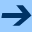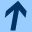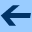On covariant embeddings of a linear functional equation with respect to an analytic iteration group

## On covariant embeddings of a linear functional equation with respect to an analytic iteration group

Jointly written with LUDWIG REICH. International Journal of Bifurcation and Chaos (World Scientific Publishing Co.) Vol. 13, No. 7, 1853 - 1875, 2003.

Abstract: Let a(x), b(x), p(x) be formal power series in the indeterminate x over ℂ (i.e. elements of the ring C[[x]] of such series), such that orda(x)=0, ordp(x)=1 and p(x) is embeddable into an analytic iteration group (π(s,x))s ∈ ℂ in C[[x]]. By a covariant embedding of the linear functional equation

(L) φ(p(x))=a(x)φ(x)+b (x),

(for the unknown series φ(x) inC[[x]] ) with respect to (π(s,x))s ∈ ℂ we understand families (α(s,x))s ∈ ℂ and (β(s,x))s ∈ ℂ

with entire coefficient functions in s, such that the system of functional equations and boundary conditions

(Ls) φ(π(s,x))=α(s,x)φ(x)+β(s,x)
(Co1) α(t+s,x)= α(s,x)α(t,π(s,x))
(Co2) β(t+s,x)= β(s,x)α(t,π(s,x)) +β(t,π(s,x))
(B1) α(0,x)=1         β(0,x)=0
(B2) α(1,x)=a(x)         β(1,x)=b(x)

holds for all solutions φ(x) of (L) and s,t in ℂ. In this paper we solve the system ( (Co1),(Co2)) (of so called cocycle equations) completely, describe when and how the boundary conditions (B1) and (B2) can be satisfied and present a large class of equations (L) together with iteration groups (π(s,x))s ∈ ℂ for which there exist covariant embeddings of (L) with respect to (π(s,x))s ∈ ℂ.

harald.fripertinger "at" uni-graz.at, March 4, 2019